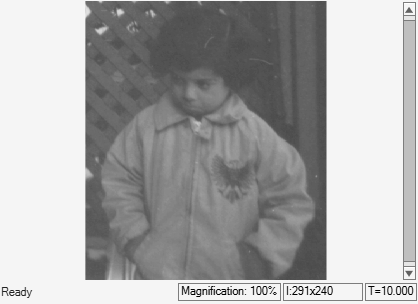# Enhance Contrast of Grayscale Image Using Histogram Equalization

This example shows how to enhance the contrast of a grayscale image using the Histogram Equalization block.

### Example Model

```modelname = 'ex_blkHistEq.slx'; open_system(modelname) ```The model reads an input image using the Image From File block. The input image in this example has very low contrast. To enhance the contrast of the input image, the model uses the Histogram Equalization block with these default parameters:

• Target Histogram: `Uniform`

• Number of bins: `64`

### Simulate and Display Results

Run the model and verify the change in contrast from the input image to the output image. For comparison, the model displays the input image and the output image using Video Viewer blocks.

```sim(modelname); ```